### Home > CALC > Chapter 6 > Lesson 6.2.2 > Problem6-77

6-77.
1. The graph of y =is shown below. The tangent line at (2, 0.5), along with the coordinate axes, form a shaded triangle. Homework Help ✎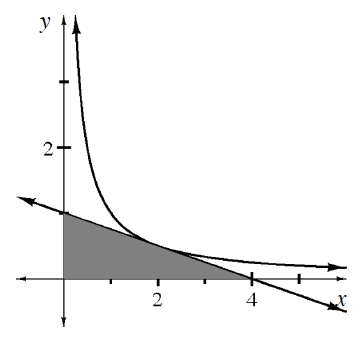1. Find the equation of the tangent line.

2. Explain why the tangent line will always give an under approximation of the curve using the second derivative.

3. Find the area of the shaded triangle.

4. Find the area of the triangle formed when the tangent is instead placed at (1, 1).

5. Prove that the area of the shaded triangle formed by a tangent to y =is always the same, regardless of the point of tangency.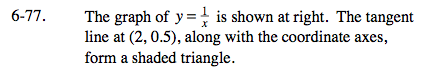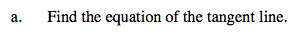Find y' and use point slope form to find the equation.

Point slope form: y = m(xx1) + y1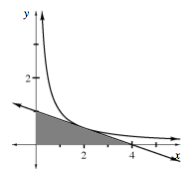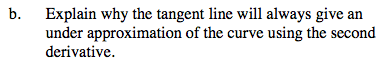If the graph was concave down, would the tangent line be an over or under-approximation?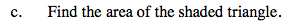The base and height are given on the graph.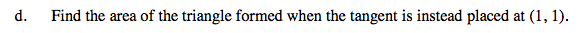Compute y'(1) and use point slope form again to find the equation.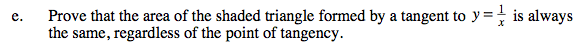$\text{For a point }\left (a, \frac{1}{a} \right ),\text{use the result of } y'(a) \text{ and point slope form to find the equation of the tangent line.}$

The vertices of the triangle made by the tangent line are (0, 0), (0, t(0)), and (x-intercept of t(x), 0), where t(x) is the equation of the tangent line.

Calculate the area of the triangle using the vertices to determine the dimensions.